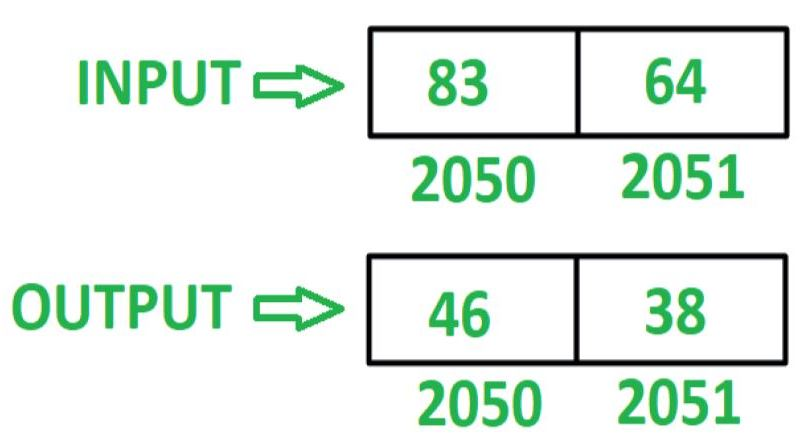GFG App
Open AppBrowser
Continue

# 8085 program to reverse 16 bit number

Problem – Write an assembly language program in 8085 microprocessor to reverse 16 bit number.

Example – Assume 16 bit number is stored at memory location 2050 and 2051.Algorithm –

1. Load contents of memory location 2050 in register L and contents of memory location 2051 in register H
2. Move contents of L in accumulator A
3. Reverse the contents of A by executing RLC instruction 4 times
4. Move the contents of A in L
5. Move the contents of H in A
6. Reverse the contents of A by executing RLC instruction 4 times
7. Move the contents of L in H
8. Move the contents of A in L
9. Store the content of L in memory location 2050 and contents of H in memory location 2051

Program –

2000 LHLD 2050 L <- M, H <- M
2003 MOV A, L A <- L
2004 RLC Rotate accumulator content left by 1 bit without carry
2005 RLC Rotate accumulator content left by 1 bit without carry
2006 RLC Rotate accumulator content left by 1 bit without carry
2007 RLC Rotate accumulator content left by 1 bit without carry
2008 MOV L, A L <- A
2009 MOV A, H A <- H
200A RLC Rotate accumulator content left by 1 bit without carry
200B RLC Rotate accumulator content left by 1 bit without carry
200C RLC Rotate accumulator content left by 1 bit without carry
200D RLC Rotate accumulator content left by 1 bit without carry
200E MOV H, L H <- L
200F MOV L, A L <- A
2010 SHLD 2050 M <- L, M <- H
2013 HLT END

Explanation – Registers A, H, L are used for general purpose.

1. LHLD 2050: loads contents of memory location 2050 in L and 2051 in H.
2. MOV A, L: moves content of L in A.
3. RLC: shift the content of A left by one bit without carry. Repeat the current instruction 4 times so that contents of A get reversed.
4. MOV L, A: moves the content of A in L.
5. MOV A, H: moves the content of H in A.
6. RLC: shift the content of A left by one bit without carry. Repeat the current instruction 4 times so that contents of A get reversed.
7. MOV H, L: moves the content of L in H.
8. MOV L, A: moves the content of A in L.
9. SHLD 2050: stores the content of L in 2050 and H in 2051.
10. HLT: stops executing the program and halts any further execution.
My Personal Notes arrow_drop_up